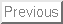Nuclear time-reversal violation and the Schiff moment of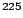Ra

J. Dobaczewski
Department of Physics and Astronomy, University of Tennessee, Knoxville, TN 37996
Physics Division, Oak Ridge National Laboratory, P.O. Box 2008, Oak Ridge, TN 37831
Joint Institute for Heavy-Ion Research, Oak Ridge, TN 37831
Institute of Theoretical Physics, Warsaw University, ul. Hoza 69, 00-681 Warsaw, Poland

J. Engel
Department of Physics and Astronomy, CB3255, University of North Carolina, Chapel Hill, NC 27599-3255

Abstract
We present a comprehensive mean-field calculation of the Schiff moment of the nucleusRa, the quantity which determines the static electric dipole moment of the corresponding atom if time-reversal (T) invariance is violated in the nucleus. The calculation breaks all possible intrinsic symmetries of the nuclear mean field and includes, in particular, both exchange and direct terms from the full finite-range T-violating nucleon-nucleon interaction, and the effects of short-range correlations. The resulting Schiff moment, which depends on three unknown T-violating pion-nucleon coupling constants, is much larger than in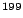Hg, the isotope with the best current experimental limit on its atomic electric-dipole moment.

The Standard Model of particle physics violates time-reversal (T) invariance, but apparently only through a single phase in the Cabibo-Kobayashi-Maskawa matrix that mixes quark flavors. The resulting T violation in flavor-conserving observables is therefore very weak and static electric-dipole moments (EDMs) of neutrons, electrons, or atoms, all of which are nonzero if T is violated, have never been observed. Standard-Model T violation is also too weak to account for the baryon asymmetry of the universe, which must come from as yet undiscovered physics. Happily, most theories of what lies beyond the Standard Model contain enough phases that flavor-conserving T violation will be unsuppressed. Current levels of sensitivity in EDM experiments are already sufficient to rule out or pressure many extra-Standard models, and it seems quite possible that with slightly improved sensitivity, new T-violating physics will be discovered.

Some of the tightest constraints on T violation come from atomic EDM experiments. The best of these at present is an experiment  withHg, but it has become clear recently [2,3,4] that atoms with octupole-deformed nuclei are potentially more sensitive than Hg. The primary reason is that given any T violation in the nucleon-nucleon interaction, an asymmetric nuclear shape and an associated parity doubling create a collective Schiff'' moment, a kind of radially weighted dipole moment (see below). Because of screening by atomic electrons, the Schiff moment, rather than the nuclear EDM, is the quantity that directly induces an atomic EDM (at least in lowest order; see Ref. ). In nuclei with symmetric shapes, a collective contribution to the Schiff moment develops only in fluctuations around that shape .

In this paper we calculate the Schiff moment ofRa, or more precisely, its dependence on any T-violating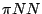couplings, in a mean-field theory that allows us to break all possible symmetries, consider a variety of phenomenologically successful strong (Skyrme) interactions, implicitly include the RPA polarization of the even-even core by the valence neutron, treat both the direct and exchange parts of the full pion-mediated interaction responsible for creating the Schiff moment, and include short-range two-body correlations between nucleons that modify the effects of this T-violating interaction. Though further refinements are possible, they will probably have to include correlations beyond mean-field theory and/or careful and systematic work on Skyrme functionals; the results presented here will not be easy to supersede.

Simpler calculations of Schiff moments have been attempted before. Ref.  applied an independent-particle model inHg,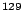Xe, and other symmetrically deformed or spherical isotopes. Refs. [8,9] carried out a much more sophisticated RPA-based calculation inHg; it's main drawback was the use of a single phenomenological interaction that made it difficult to estimate uncertainty. Ref.  made estimates in a particle-rotor model of the enhancement due to octupole deformation, and in Ref.  we applied a preliminary version of our technique toRa, an experiment on which is in the works . That paper, however, assumed the range of the T-violating interaction to be zero, an especially bad approximation for exchange matrix elements, and was unable (obviously) to examine the effects of short-range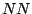correlations.

We briefly review some definitions and ideas. The Schiff moment is given by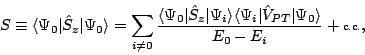(1)

where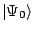is the member of the ground-state multiplet with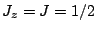(positive parity), the sum is over excited states, and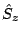is the operator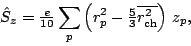(2)

with the sum here over protons, and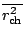the mean-square charge radius. The operator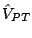in Eq. (1) is the T- (and parity-) violating nucleon-nucleon interaction mediated by the pion [2,12]: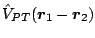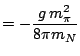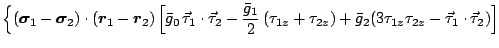(3)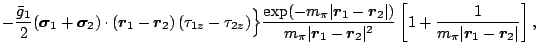where arrows denote isovector operators,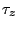is +1 for neutrons,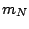is the nucleon mass, and (in this equation only) we use the convention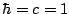. The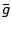's are the unknown isoscalar, isovector, and isotensor T-violating pion-nucleon coupling constants, and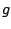is the usual strongcoupling constant.

The asymmetric shape ofRa implies parity doubling (see e.g. Ref. ), i.e. the existence of a very low-energy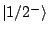state, in this case 55keV  above the ground state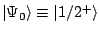, that dominates the sum in Eq. (1) because of the corresponding small denominator. With the approximation that the shape deformation is rigid, the ground state and its negative-parity partner in octupole-deformed nucleus are projections onto good parity and angular momentum of the same intrinsic state" (see Fig. 1), which represents the wave function of the nucleus in its own body-fixed frame with the total angular momentum aligned along the symmetry axis. Equation (1) then reduces to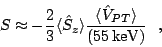(4)

where the brackets indicate expectation values in the intrinsic state. Using Eq. (3) for, we can express the dependence of the Schiff moment on the undetermined T-violatingvertices as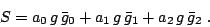(5)

The coefficients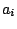, which are the result of the calculation, have units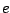fm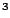.

The octupole deformation enhances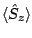, the first factor in Eq. (4), making it collective, robust, and straightforward to calculate with an error of a factor of two or less. The interaction expectation value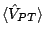is harder to estimate because it is sensitive to the nuclear spin distribution, which depends on delicate correlations near the Fermi surface. Our calculation allows the breaking of Kramers degeneracy in the intrinsic frame and, consequently, spin polarization.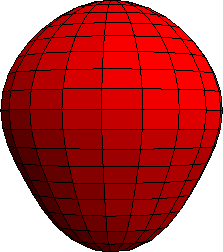To evaluatewe constructed a new version of the code HFODD (v2.14e) [15,16]. The code uses a triaxial harmonic-oscillator basis and Gaussian integration to solve self-consistent mean-field equations for zero-range Skyrme interactions. Evaluating matrix elements of the finite-range interaction (3) is much harder numerically, but efficient techniques have already been developed  for Gaussian interactions, which are separable in three Cartesian directions. The spatial dependence in Eq. (3) is different, the derivative of a Yukawa function, and we also include short-range correlations between nucleons (which the mean-field does not capture) by multiplying the interaction by the square of a correlation function  that cuts off the two-nucleon wave functions below a relative distance of about a fermi: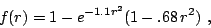(6)

with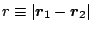in fermis and the coefficients of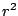in fm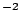. The resulting product looks very different from a Gaussian, but we were able to reproduce it quite accurately (see Fig. 2) with the sum of four Gaussians: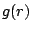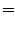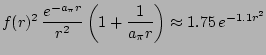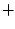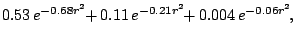(7)

where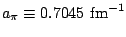is the pion mass in inverse fermis and the numbers in the fit all have units fm. The extra factor of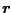not included in Eq. (7) (i.e. the factor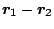in Eq. (3)) is treated separately.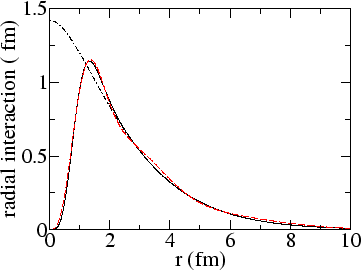HFODD works with any Skyrme energy functional. In the context of the present study the best is SkO[19,20]. The time-even'' terms in this interaction, which act in even-nucleus ground states, were fit with special attention to nuclei around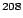Pb and to spin-orbit splitting. The time-odd'' terms responsible for core-polarization in an odd nucleus were adjusted in Ref.  to reproduce Gamow-Teller resonances, resulting in an effective Landau parameter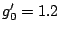. (The isoscalar parameter was set to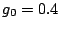, following common practice.) For comparison, we also carry out the calculation with the older parameterizations SLy4, SIII, and SkM* (with time-odd terms that are not fixed by gauge invariance neglected, and then again, with the simplest time-odd terms modified so that the Landau parameters have the same values as in SkO) but have the most confidence in SkO. Ref.  presented predictions by these functionals for the binding energies, separation energies, intrinsic dipole moments, and spin-orbit splittings in the even Ra isotopes. SkOand SIII seemed to do the best job.

Table 1 shows the calculated value, with SkO, of the three coefficientsat several levels of approximation. The finite range reduces the direct matrix elements of the interaction (and the corresponding) from the zero-range limit  significantly. The exchange terms are reduced much more, so that they are always smaller than the direct terms. The effects of the short-range correlations, which also reduce the coefficients, are relatively small as well but non-negligible. The coefficients produced by SIII are similar to those in the table, while the other two forces give numbers that are larger by factors of two or three, whether or not the Landau parameters are adjusted, i.e., the effects of adjusting those parameters seem to be fairly small. Finally, as pointed out in Ref. , relativistic effects in electron wave functions correct the effects of the Schiff moment; the authors summarize the corrections in a quantity the call the local nuclear dipole moment''. Our local dipole moment inRa is 82% of the Schiff moment for the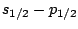atomic transition and 86.5% for the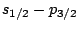transition.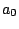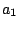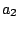zero-range (direct only)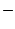5.1 10.410.1 finite-range (direct only)1.9 6.33.8 finite-range + src (direct only)1.7 6.03.5 finite-range + src (direct + exchange)1.5 6.04.0

What is the uncertainty in our numbers? The mean-field omits correlations that could have some effect on the result; those could be explored by refining the calculation through angular-momentum and parity projection, i.e., the restoration of symmetries broken by the mean field. In addition, an optimal Skyrme functional has yet to be identified. Those we tested give results that differ from the SkOnumbers by factors of up to two or three, as mentioned above. But some low-order terms in the T-odd part of the Skyrme functional are never used even in SkO, because they have never been fit. Ref.  constrained some combinations of those terms but others were set to zero for lack of sufficient Gamow-Teller data in spherical nuclei. One might imagine trying to fit in deformed nuclei, or looking at spin-strength distributions with different total angular momentum and parity; the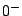channel would be particularly useful because those are the quantum numbers of. At the same time, it would probably help to explicitly study the sensitivity of the Schiff moments to changes in the various Skyrme parameters, both in the time-odd and time-even sectors. With enough work on all these fronts we could give a firmer estimate of the uncertainty than our current guess: a factor of two or three.

If we accept our current results as reasonably accurate, we are in a position to quantify the advantages ofRa for an EDM measurement. A recent RPA calculation [8,9] of theforHg gives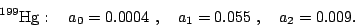(8)

Our numbers are more than two orders of magnitude larger, particularly in the isoscalar channel (the relevant channel if T-violation is caused by a nonzero QCD parameter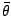), where the Hg number is anomalously small. Atomic physics enhances any EDM in Ra by another factor of 3 over that in Hg , so if the Ra EDM can be measured even 1/100th as accurately as that of Hg, the sensitivity to nuclear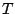violation will be significantly greater.

In conclusion, we have evaluated the Schiff moment ofRa in a completely symmetry-breaking mean-field approach, including in particular the finite-range matrix elements of the T-violating nucleon-nucleon interaction. The results indicate that EDM experiments in this system are very promising. The remaining uncertainties of a factor of two or three are related primarily to deficiencies in nuclear effective interactions, which can be removed but not easily.

Fruitful discussions with W. Nazarewicz are gratefully acknowledged. This work was supported in part by the U.S. Department of Energy under Contracts Nos. DE-FG02-96ER40963 (University of Tennessee), DE-AC05-00OR22725 with UT-Battelle, LLC (Oak Ridge National Laboratory), DE-FG05-87ER40361 (Joint Institute for Heavy Ion Research), DE-FG02-97ER41019 (University of North Carolina); by the Polish Committee for Scientific Research (KBN) under Contract No. 1 P03B 059 27; and by the Foundation for Polish Science (FNP).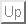Next: Bibliography
Jacek Dobaczewski 2005-03-25